×
INTELLIGENT WORK FORUMS
FOR ENGINEERING PROFESSIONALS

Are you an
Engineering professional?
Join Eng-Tips Forums!
• Talk With Other Members
• Be Notified Of Responses
• Keyword Search
Favorite Forums
• Automated Signatures
• Best Of All, It's Free!

*Eng-Tips's functionality depends on members receiving e-mail. By joining you are opting in to receive e-mail.

#### Posting Guidelines

Promoting, selling, recruiting, coursework and thesis posting is forbidden.

# Calculate Section Modulus for an Irregular Section - (using X, Y Cordinates)7

## Calculate Section Modulus for an Irregular Section - (using X, Y Cordinates)

(OP)
Hi all,

I have a simple mechanics of solids type question which I have been googling, but I have not been able to find an answer.
I have an irregular shaped section with bending moments imposed about its X axis as shown below.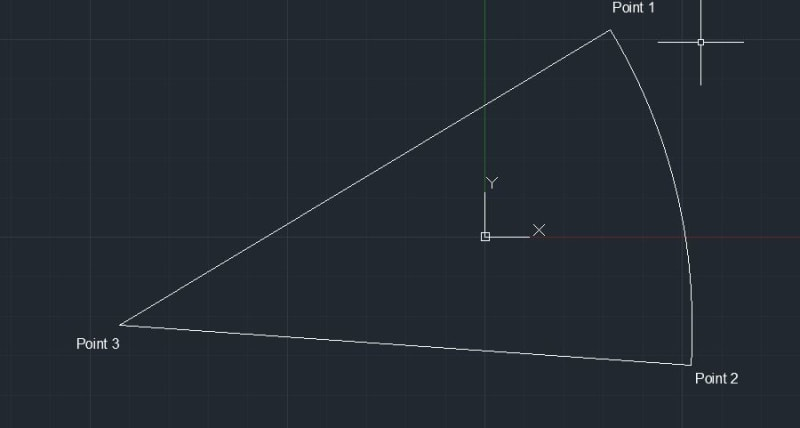The centroid of the shape coincide with the x=0 aand y = 0 of the co-ordinate system.

Now for me to check my bending stresses at point 1 , 2 and 3, I need calculate my section modulus about Section X for each of these points.
If this was a simple rectangle, we could simply use B * H^2/6.

Is there any formular which would let me calculate the corresponding section modulus for these three points if I provide co-ordinates and Ix, Iy and Ixy values which I cam get of Autocad.

For context, I am trying model a deteriorated timber pile to check load capacity.

Any help would be appreciated.

Cheers,
Dimuth

### RE: Calculate Section Modulus for an Irregular Section - (using X, Y Cordinates)

Can you find the results for a segment, with the centroid located parallel to the outer part of the circle, and rotate the values? I seem to recall there was a transformation to the I value based on rotation...

Rather than think climate change and the corona virus as science, think of it as the wrath of God. Feel any better?

-Dik

### RE: Calculate Section Modulus for an Irregular Section - (using X, Y Cordinates)

Here's the first part...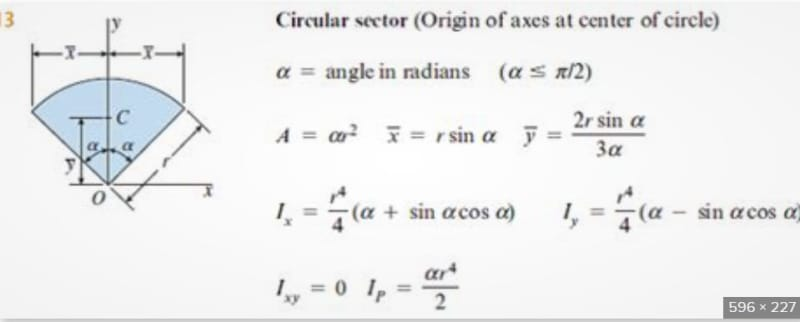and maybe the second...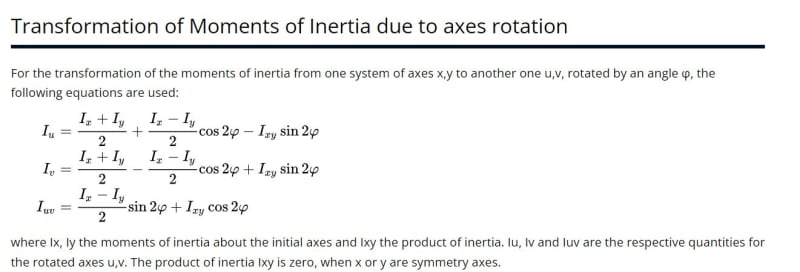I think that will do it... just a matter of putting the stuff together, I think. That goes back so far, it makes my head hurt... over 50 years. Having the new moments of inertia, it's a matter of determining the Section Modulii. Let me know if it works.Rather than think climate change and the corona virus as science, think of it as the wrath of God. Feel any better?

-Dik

### RE: Calculate Section Modulus for an Irregular Section - (using X, Y Cordinates)

https://newtonexcelbach.com/2018/06/24/using-secti...
will find section properties for a variety of defined shapes.

The standard shape for a circular segment is symmetrical about the x-axis, but the group feature allows you to rotate any shape about the origin, which should give the results you need. The link above gives an example of rotating a circular segment through 90 degrees, but you can use any angle.

Alternatively (and the way I'd probably go) you can define the shape with coordinates and get the section properties for any shape.

Doug Jenkins
Interactive Design Services
http://newtonexcelbach.wordpress.com/

### RE: Calculate Section Modulus for an Irregular Section - (using X, Y Cordinates)

(OP)
Thanks Dik, for your input, I am still missing the vital bit I need to get the section modulus.
I may have to go for the old method of picking Ixx / distance from centroid to extreme comrpession/tension fiber, <- and guess the extreme points

### RE: Calculate Section Modulus for an Irregular Section - (using X, Y Cordinates)

Just tried the spreadsheet, and it seems to work OK:

On the DefShapes sheet, select "Circular Sector" and enter the radius and theta: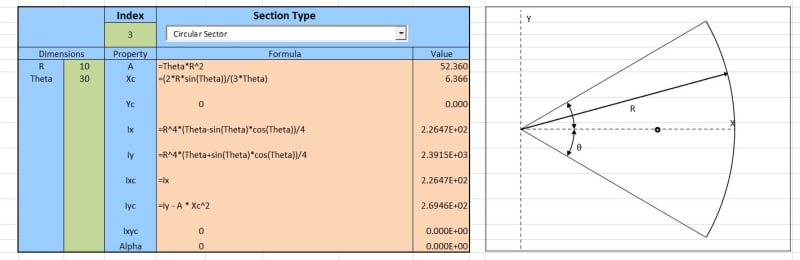Scroll down to the Group properties input, enter -Xcentroid as "X offset" and the rotation angle, then click "Create New Group":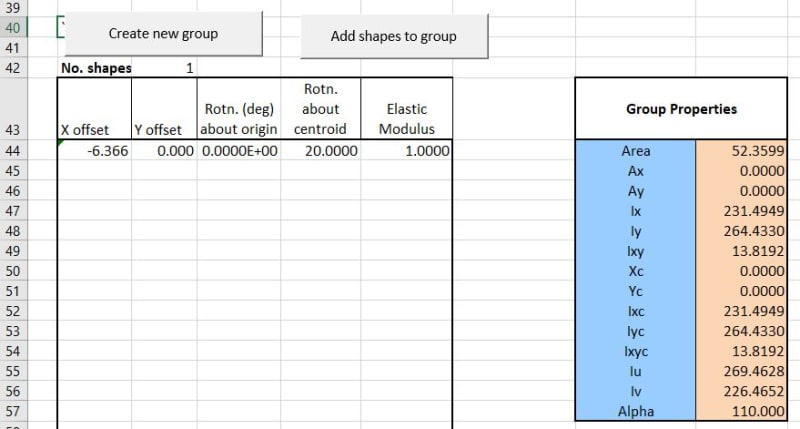Go to the Coords_Group sheet and click Plot Group. You can then find the coordinates of the three corner points, with the origin at the centroid, which you can use to calculate the 3 section modulus values: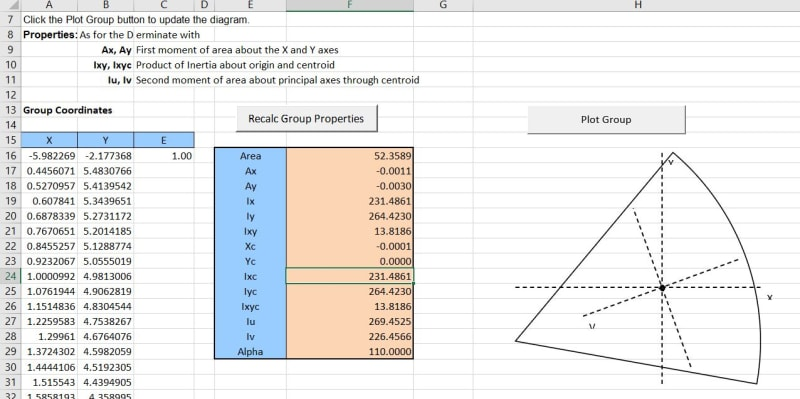Doug Jenkins
Interactive Design Services
http://newtonexcelbach.wordpress.com/

### RE: Calculate Section Modulus for an Irregular Section - (using X, Y Cordinates)

do you want a sector, which is easily done, or a generalised shape ?

you can always go back to the basic definition of moments of area ... intergral of xdA and integral of x^2dA.
or you can divide your shape into smaller standard pieces (squares and triangles) and sum.

### RE: Calculate Section Modulus for an Irregular Section - (using X, Y Cordinates)

If you have the point coords, you have the geometry. For that type of shape, I'd draw the object in autocad and measure the angles and dimensions. Draw it, find the centroid, draw the centroid, and take measurements, rotate it and take new measurements.

Rather than think climate change and the corona virus as science, think of it as the wrath of God. Feel any better?

-Dik

### RE: Calculate Section Modulus for an Irregular Section - (using X, Y Cordinates)

CAD should do it all for you, no?

### RE: Calculate Section Modulus for an Irregular Section - (using X, Y Cordinates)

You should probably also allow for the fact that, as per your diagram at least, your "pile" cross-section is not being bent about a principal axis.  (Inverted commas because that's an impressive amount of deterioration you seem to be showing.)

### RE: Calculate Section Modulus for an Irregular Section - (using X, Y Cordinates)

Thanks, Doug...

Rather than think climate change and the corona virus as science, think of it as the wrath of God. Feel any better?

-Dik

### RE: Calculate Section Modulus for an Irregular Section - (using X, Y Cordinates)

In addition to the other solutions noted, numerical integration via spreadsheet is fairly easy to whip up. Presumably, you don't know the geometry to 4 decimal places anyway, so it wouldn't be hard to get close enough.

### RE: Calculate Section Modulus for an Irregular Section - (using X, Y Cordinates)

Am I missing something? You state you have Ix and Iy. The stress at a given point for a moment about the x-axis is then Fb=(M Y)/Ix. Fb is the stress, M is the moment, Y is the perpendicular distance from the X-axis to the point under consideration.

### RE: Calculate Section Modulus for an Irregular Section - (using X, Y Cordinates)

So then it’s a matter of determining the moments of inertia about the principal axes per DIK’e post and resolving the moment to the components about the principal axes, no?

### RE: Calculate Section Modulus for an Irregular Section - (using X, Y Cordinates)

I'm pretty sure of it... and then S can be determined by the x and y distance to the outside fibre. If I'm bored tomorrow, I'll do up an SMath program. Nearly finished my lag screw one, but want to check the output with an actual connection design program. I don't have one, but my colleague Vic has one... he's the wood expert I use.

Rather than think climate change and the corona virus as science, think of it as the wrath of God. Feel any better?

-Dik

### RE: Calculate Section Modulus for an Irregular Section - (using X, Y Cordinates)

Something like (ERROR: Iy HAS TO BE TRANFORMED):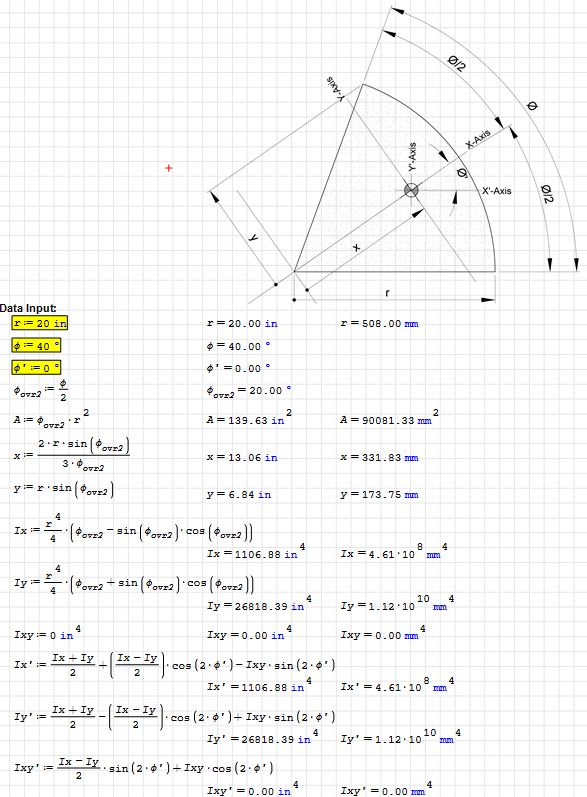Rather than think climate change and the corona virus as science, think of it as the wrath of God. Feel any better?

-Dik

### RE: Calculate Section Modulus for an Irregular Section - (using X, Y Cordinates)

Ixy = 0 is surprising!

Edit: See below!

BA

### RE: Calculate Section Modulus for an Irregular Section - (using X, Y Cordinates)

#### Quote (Ixy = 0 is surprising!)

I guess the formula's correct... I didn't check the derivation... just plugged numbers into a formula... and if you set the angle of rotation equal to 0, you get the same values for no rotation... something seems to be OK.

Rather than think climate change and the corona virus as science, think of it as the wrath of God. Feel any better?

-Dik

### RE: Calculate Section Modulus for an Irregular Section - (using X, Y Cordinates)

I spoke too soon. It is not surprising, because the shape is symmetrical about the X axis. Sorry about that, dik.

BA

### RE: Calculate Section Modulus for an Irregular Section - (using X, Y Cordinates)

dik - with inch units and principal axis parallel to the X axis, and origin at the centre of the circle I get: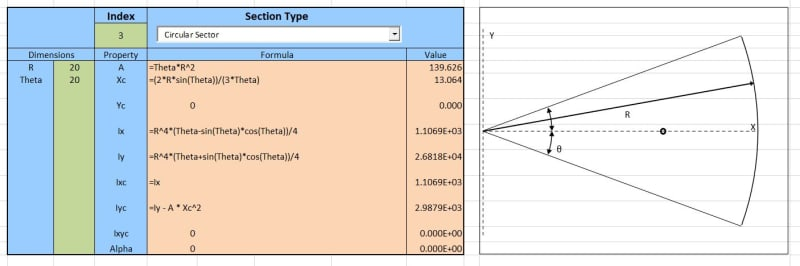Rotating the axis by 20 degrees and moving the origin to the CoG I get: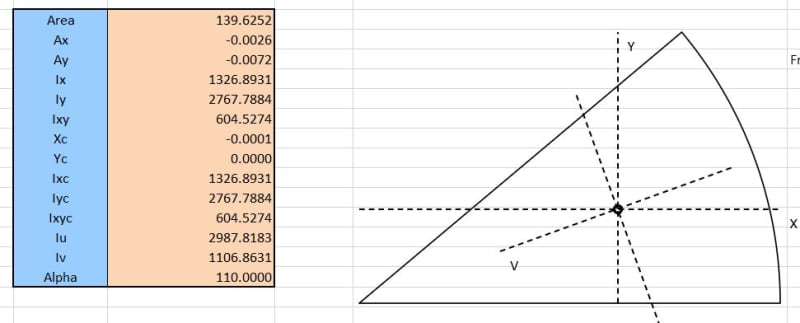Note that your Iy seems to be with the Y axis through the circle centre, and your Iy' has the same value, but should be much smaller.
Your Ix' agrees with my Iv.
You have zero for Ixy' but it should be non-zero for non-principal axes.

Note that my second screenshot uses point coordinates with a large number of segments for the curve; hence the small non-zero values for the centroid and first moments of area.

Also I think we are over-complicating this. Why not find the section properties and section modulus values with the principal axes parallel to horizontal and vertical, then rotate the applied moments, rather than rotating the cross section?

Note:
So we agree that we don't need to rotate the section, but the Iy does need to be with the Y axis through the centroid (i.e. the IYc value in my first screenshot).

Doug Jenkins
Interactive Design Services
http://newtonexcelbach.wordpress.com/

### RE: Calculate Section Modulus for an Irregular Section - (using X, Y Cordinates)

@dik,

Iy/Ix = 24.3 seems wrong.

Ixy = 0 is okay when X axis is an axis of symmetry.

The primed values, Ix', Iy' and Ixy' can't be equal to the non-primed values, Ix, Iy and Ixy.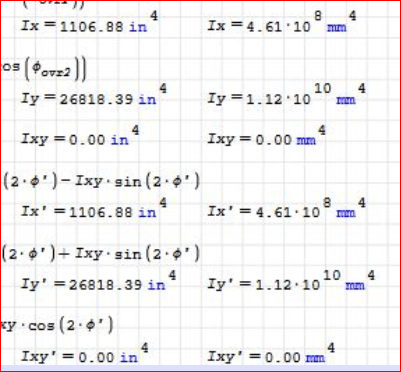BA

### RE: Calculate Section Modulus for an Irregular Section - (using X, Y Cordinates)

(ERROR: Iy HAS TO BE TRANSFORMED) They should be identical if the angle of rotation is 0 deg/rad. It was my only quick check... I didn't check the initial formulae.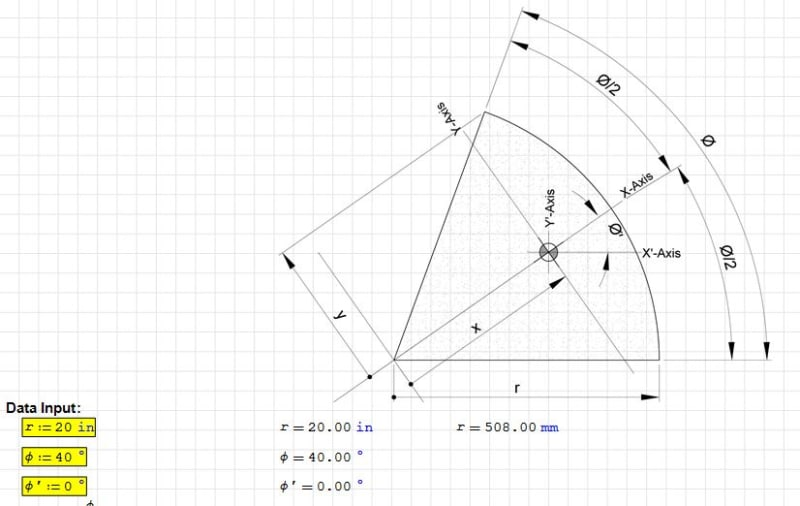Rather than think climate change and the corona virus as science, think of it as the wrath of God. Feel any better?

-Dik

### RE: Calculate Section Modulus for an Irregular Section - (using X, Y Cordinates)

dik - could you post a summary of the results for the non-SMath users.

Doug Jenkins
Interactive Design Services
http://newtonexcelbach.wordpress.com/

### RE: Calculate Section Modulus for an Irregular Section - (using X, Y Cordinates)

(ERROR: Iy HAS TO BE TRANSFORMED) Not much to it... attached. If you're not running SMath... you should. Other than a couple of minor hiccups, it's a great free program and really useful.
Only downside is political and that it was written by a Russian.

Rather than think climate change and the corona virus as science, think of it as the wrath of God. Feel any better?

-Dik

### RE: Calculate Section Modulus for an Irregular Section - (using X, Y Cordinates)

Thanks dik. I probably do have SMath installed somewhere, but I prefer the spreadsheet style interface, so I'll stick wit Excel.

Doug Jenkins
Interactive Design Services
http://newtonexcelbach.wordpress.com/

### RE: Calculate Section Modulus for an Irregular Section - (using X, Y Cordinates)

I use both... for AISC steel sections database and stuff related to it, Excel cannot be beat. I like SMath because of the formulas and the units.

Rather than think climate change and the corona virus as science, think of it as the wrath of God. Feel any better?

-Dik

### RE: Calculate Section Modulus for an Irregular Section - (using X, Y Cordinates)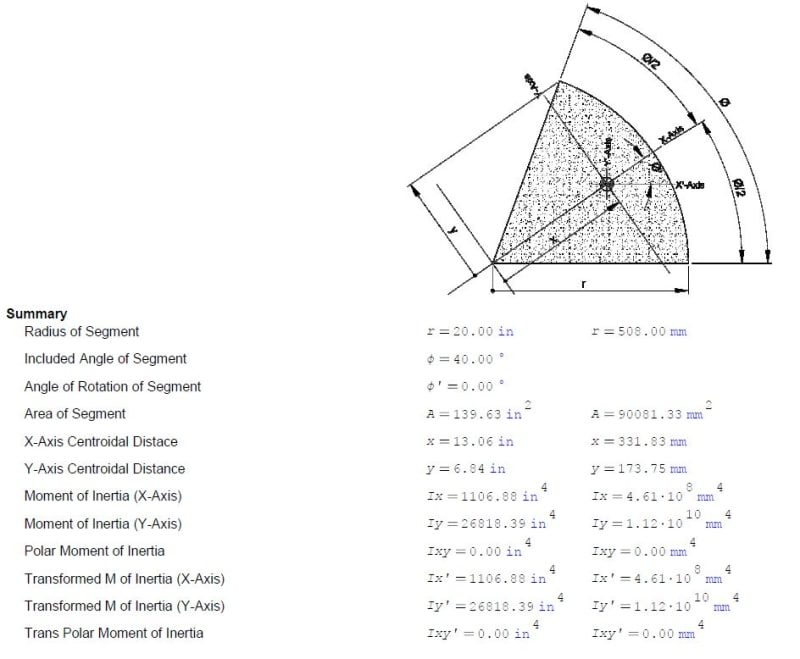With the origin at the centre of the circle and X axis passing through the centroid I agree with your Ix, Iy and Ixy values, and X axis centroidal distance, but:
Yc should be 0
For Theta' = 0 the Ix' and Ixy' are unchanged, but Iy' should be much smaller.

I get: Iy' = 2987.p in^4

Also the diagram is confusing, but I am assuming for Theta' = 0 the transformed axes should be parallel to the original axes.

Doug Jenkins
Interactive Design Services
http://newtonexcelbach.wordpress.com/

### RE: Calculate Section Modulus for an Irregular Section - (using X, Y Cordinates)

With phi primed equal to zero, there is absolutely no change to the original x and y axis configuration so all aspects of Ix', Iy' and Ixy' should be identical to the Ix, Iy and Ixy values. That's correct... rotation if any would be about the centroid. The only thing I checked was that with the x value calculated... the y value is consistent.

Rather than think climate change and the corona virus as science, think of it as the wrath of God. Feel any better?

-Dik

### RE: Calculate Section Modulus for an Irregular Section - (using X, Y Cordinates)

But the prime coordinates have their origin at the centroid rather than the centre of the circle, so Iy' should be different even if the X axis is unchanged.

Doug Jenkins
Interactive Design Services
http://newtonexcelbach.wordpress.com/

### RE: Calculate Section Modulus for an Irregular Section - (using X, Y Cordinates)

My error... I just realised the coordinates in the original drawing were at the centre of the circle... and not at the centroid. The Iy has to be corrected for the (area * x^2). Thanks, My apologies...

Rather than think climate change and the corona virus as science, think of it as the wrath of God. Feel any better?

-Dik

### RE: Calculate Section Modulus for an Irregular Section - (using X, Y Cordinates)

How are X and Y-Axis Centroidal Distance defined?

If the origin of the original section is at the centroid, shouldn't they both be zero?

Doug Jenkins
Interactive Design Services
http://newtonexcelbach.wordpress.com/

### RE: Calculate Section Modulus for an Irregular Section - (using X, Y Cordinates)

I accidentally transformed the coordinates from the centre of the circle segment to the centroid and should have modified Iy. Segment is symmetric about the x-axis so change in Iy is simply the original Iy less the (area times the x distance squared). Ix doesn't change. I've noted the error in the messages above. A log of changes to the program show up in the SMath program, but not in the *.pdf file.

I think I'll go sit in the corner now and put on my pointed hat...Rather than think climate change and the corona virus as science, think of it as the wrath of God. Feel any better?

-Dik

### RE: Calculate Section Modulus for an Irregular Section - (using X, Y Cordinates)

I get the same properties as IDS, for the 20" radius and 40 degree sweep. I also have the stress worked out with general bending formula or transformation of bending moment to the principal axis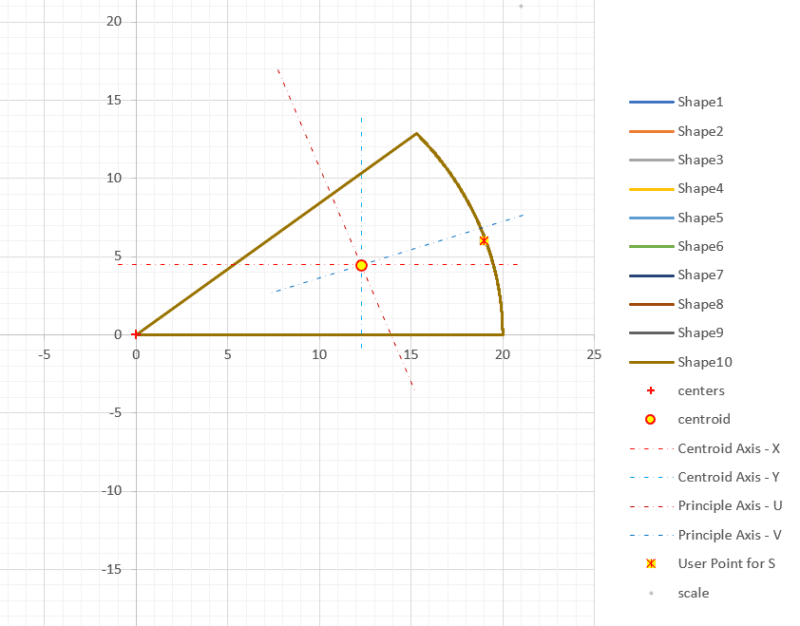:
A: 139.6263 in2
Cx: 12.2763 in
Cy: 4.4682 in

Centroidal Axis Properties Parallel to global x and y axis
Ixx: 1326.9146 in4
Iyy: 2767.8334 in4
Ixxyy: 604.5372 in4
Jzz: 4094.7480 in4
rxx: 3.0827 in
ryy: 4.4523 in
rzz: 5.4154 in

Principle Axis Properties
A 2047.3740
B -720.4594
I1 2987.8669 in4
I2 1106.8811 in4
Iuu 2987.8669 in4
Ivv 1106.8811 in4
Iuuvv 0.0000 in4
Θu -70.0000 degrees
Θv 20.0000 degrees
Jkk 4094.7480 in4
ruu 4.6259 in
rvv 2.8156 in
rkk 5.4154 in

Elastic Section Moduli
Sx+ 158.2009 in3
Sx- 296.9671 in3
Sy+ 358.3576 in3
Sy- 225.4608 in3

At User Point
x 19 in Dist. To U 6.8421 in
y 6 in Dist. To V -0.8602 in

Sx 866.2574 in3 Su 436.6901 in3
Sy 411.6556 in3 Sv -1286.7314 in3

Elastic Stresses at user point from loads applied at shape centroid
P = 0.0000 kips 0.000 lbs positive = tension
Mx = 10.0000 ft-kips 120000.000 in-lbs positive creates Tension on top surface
My = 0.0000 ft-kips 0.000 in-lbs positive creates Tension on left surface

σ,axial = P/A = 0.0000 ksi 0.0000 psi

Mx:
y (in) dy = (y-cy) (in) σy =( (Mx Iyy - My Ixxyy) / ( Ixx Iyy - Ixxyy^2) ) * dy [ General Elastic Bending Stress Formula which captures non principle axis bending, if Ixxyy = 0 reduces to the common stress formula = Mx dy /Ixx ]
6.0000 1.5318 153.8349 psi

My:
x (in) dx = (x-cx) (in) σx =( (My Ixx - Mx Ixxyy) / ( Ixx Iyy - Ixxyy^2) ) * dx [ General Elastic Bending Stress Formula which captures non principle axis bending, if Ixxyy = 0 reduces to the common stress formula = My dx /Iyy ]
19.0000 6.7237 -147.4850 psi

σ,user point = 0.0063 ksi 6.3499 psi [From General Bending Stress Formulas using Geometric Bending]

σ,user point = 0.0063 ksi 6.3499 psi [From Transformation of Bending Moments to Principal Axis]

Principal Axis Moments
Mv = 9.397 ft-kips 112763.114 in-lbs σ,v = -87.6353 psi
Mu = 3.420 ft-kips 41042.417 in-lbs σ,u = 93.9852 psi
σ,bending = 6.3499 psi

My Personal Open Source Structural Applications:
https://github.com/buddyd16/Structural-Engineering

Open Source Structural GitHub Group:
https://github.com/open-struct-engineer

### RE: Calculate Section Modulus for an Irregular Section - (using X, Y Cordinates)

Yup...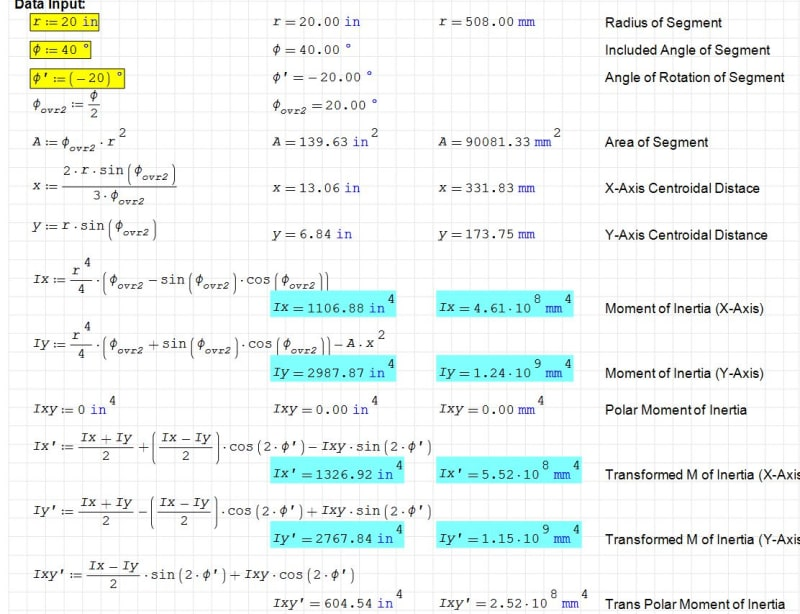Rather than think climate change and the corona virus as science, think of it as the wrath of God. Feel any better?

-Dik

### RE: Calculate Section Modulus for an Irregular Section - (using X, Y Cordinates)

Correct... will fix designation and thanks.

Rather than think climate change and the corona virus as science, think of it as the wrath of God. Feel any better?

-Dik

### RE: Calculate Section Modulus for an Irregular Section - (using X, Y Cordinates)

#### Quote (also can't have one of these threads without mentioning Handofthelion's impressive program:)

Rather than think climate change and the corona virus as science, think of it as the wrath of God. Feel any better?

-Dik

### RE: Calculate Section Modulus for an Irregular Section - (using X, Y Cordinates)

(OP)
Hi Guys thank you very much for the input, and Dik I am going to install Smath and have a look at what you did.
I have never used Smath before.

### RE: Calculate Section Modulus for an Irregular Section - (using X, Y Cordinates)

It's an excellent program... with a few little hiccups, but generally very good. I've written hundreds of programs using it... almost anything I have to design more than a couple of times, I'll do it up. Getting the hang of writing the formula is difficult to start with but after a while I can do formulae almost as fast as writing them. It keeps track of units, very well. I've attached a snapshot of my current program on lagscrews to show the complexity of the formulae. The text in blue are the units and the program takes care of them, too. Only downside to the program is that it was written by a Russian dude and that my have political fallout... I'm not concerned about such stuff. Larger programs can be written by cutting and pasting parts of other programs. Most of my programs are over 10 pages.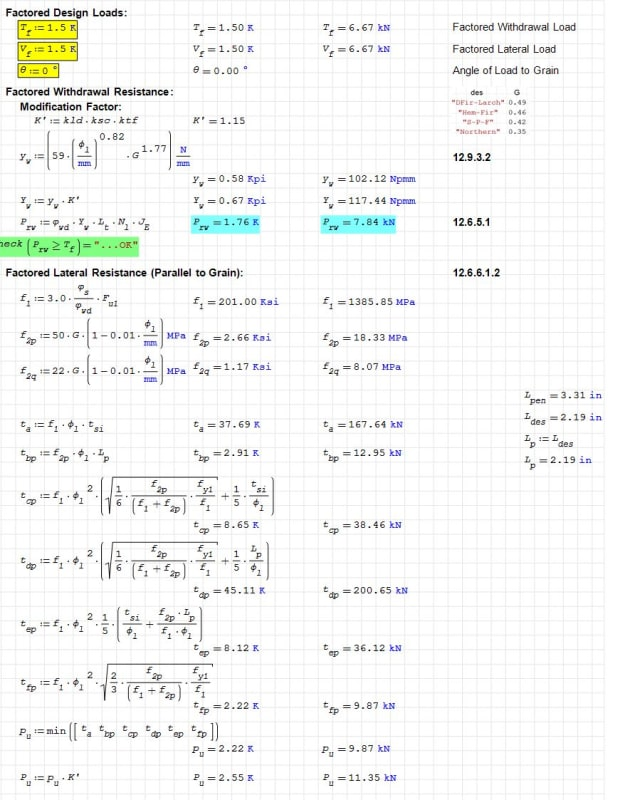Rather than think climate change and the corona virus as science, think of it as the wrath of God. Feel any better?

-Dik

### RE: Calculate Section Modulus for an Irregular Section - (using X, Y Cordinates)

Here are my stress calculations. Using the same section as before, all in inch and lbf units:

First find the offsets from the points where stresses are required to the principal axes (points 1-3 are the corners, point 4 is the point used in Celt's calcs: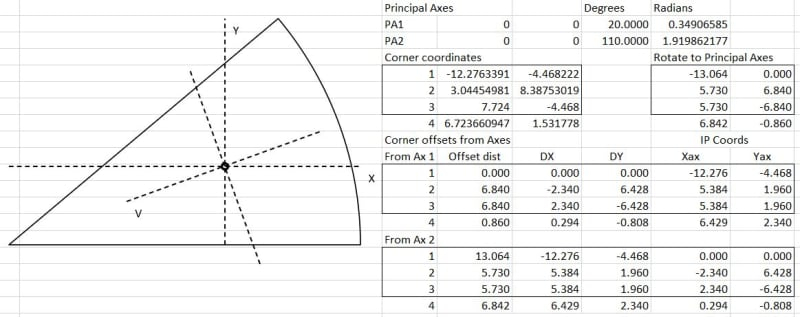Then calculate stresses using the principal axes then the global XY axes: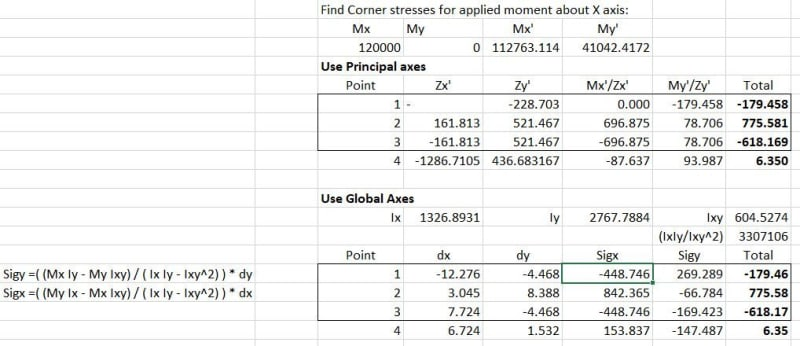So the results are consistent and also agrees with Celts results.

Having worked through the process I now think it is simplest to use the original axes with the full bending stress formulas:
Sigy =( (Mx Iy - My Ixy) / ( Ix Iy - Ixy^2) ) * dy
Sigx =( (My Ix - Mx Ixy) / ( Ix Iy - Ixy^2) ) * dx

Doug Jenkins
Interactive Design Services
http://newtonexcelbach.wordpress.com/

#### Red Flag This Post

Please let us know here why this post is inappropriate. Reasons such as off-topic, duplicates, flames, illegal, vulgar, or students posting their homework.

#### Red Flag Submitted

Thank you for helping keep Eng-Tips Forums free from inappropriate posts.
The Eng-Tips staff will check this out and take appropriate action.

#### Resources

Low-Volume Rapid Injection Molding With 3D Printed Molds
Learn methods and guidelines for using stereolithography (SLA) 3D printed molds in the injection molding process to lower costs and lead time. Discover how this hybrid manufacturing process enables on-demand mold fabrication to quickly produce small batches of thermoplastic parts. Download Now
Examine how the principles of DfAM upend many of the long-standing rules around manufacturability - allowing engineers and designers to place a partâ€™s function at the center of their design considerations. Download Now
Industry Perspective: Education and Metal 3D Printing
Metal 3D printing has rapidly emerged as a key technology in modern design and manufacturing, so itâ€™s critical educational institutions include it in their curricula to avoid leaving students at a disadvantage as they enter the workforce. Download Now

Close Box

# Join Eng-Tips® Today!

Join your peers on the Internet's largest technical engineering professional community.
It's easy to join and it's free.

Here's Why Members Love Eng-Tips Forums:

•Talk To Other Members
• Notification Of Responses To Questions
• Favorite Forums One Click Access
• Keyword Search Of All Posts, And More...

Register now while it's still free!